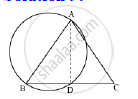Share

Show that the Circle Drawn on Any One of the Equal Sides of an Isosceles Triangle as Diameter Bisects the Base. - ICSE Class 10 - Mathematics

Question

Show that the circle drawn on any one of the equal sides of an isosceles triangle as diameter bisects the base.

SolutionAB is the diameter.
∴ ∠ADB = 90º (Angle in a semi-circle)
But, ∠ADB + ∠ADC = 180º (linear pair)
⇒ ∠ADC = 90º
In ΔABD and ΔACD,
AB = AC (Given)
ΔABD ≅ ΔACD (RHS congruence criterion)
⇒ BD = DC (C.P.C.T)
Hence, the circle bisects base BC at D.

Is there an error in this question or solution?

Video TutorialsVIEW ALL 

Solution Show that the Circle Drawn on Any One of the Equal Sides of an Isosceles Triangle as Diameter Bisects the Base. Concept: Tangent to a Circle.
S